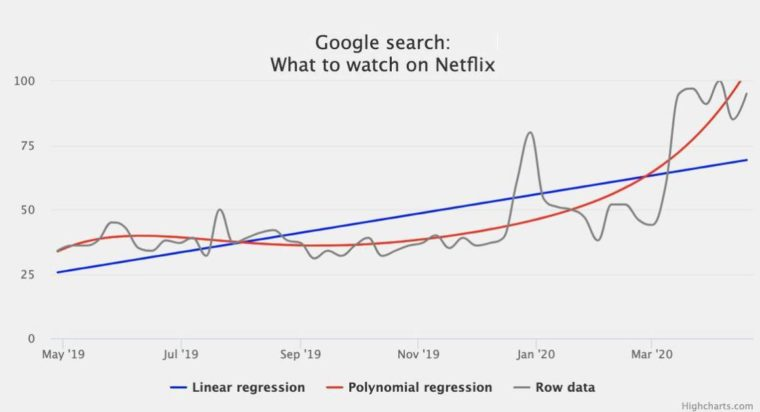# Data science and Highcharts: polynomial regressionIn this article, we will show you how to calculate and visualize polynomial regression using the regression-js library and Highcharts. We will also review the benefits and limitations of this type of regression.

Remark
The Highcharts Stock package has built-in support for advanced technical indicators including linear regressions and more. This blog article, however, focuses on how you can apply custom statistical analysis on the chart data, and render it using Highcharts.

In our previous article, we used a linear regression model to explore the relationship between the height and weight of athletes participating in the 2012 Summer Olympics. This model is simple and easy to implement and fits well with a dataset with a fairly linear relationship between the data. For a dataset that doesn’t follow a simple, predictable trend curve, we need to apply different models to illustrate the correlations, and one of them is a polynomial regression.

The demo below visualizes the worldwide trend of the query “What to watch on Netflix” between late April 2019 to the beginning of April 2020.

I had to play with the order of the equation in this line of code:

```let resultPolynomial = regression.polynomial(data, {
order: 5,
precision: 20
});```

until I realized that the 5th order produces the right model to fit the data. So the equation looks like the following:

```y = 0.00000273707499263095 x ^ 5 + -0.000345103845158… 154 x ^ 2 + 3.0846793394495107 x + 31.035997607315213
```

From the row data representation (in the gray color), we can see that there is a progression in the number of searches of the phrase “What to watch on Netflix.” The linear regression model (in blue) follows the progress, but it doesn’t fit well the row data. In this case, the regression line model underfits the data, where the polynomial regression (in red) model fits much better. Our polynomial regression model is much balanced.

Even though the polynomial regression technique seems to be perfect for modeling the relationship between variables, you have to be careful to select the right order and avoiding the overfitting situation. The demo below displays the same data “What to watch on Netflix” during the same period, the only difference is that the polynomial model has an order of 10, and it fits too well the data. Such a model could not work well on the test or real data sets as it is too much personalized with the actual data:

By now, you are well equipped to use Highcharts with any statistical library to create linear and polynomial regression models.

Feel free to come up with your own statistical project visualization and share your experience or questions in the comment section below.

In our next post, we will look at how to use the statistical tools that are already included in Highcharts Stock.